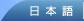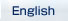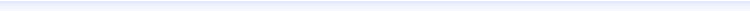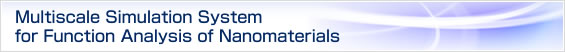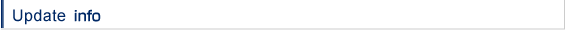PHASE

Using PHASE, electronic states of matters are calculated based on the density functional theory.

What can be calculated by using PHASE?

• total energy
• charge density distribution
• electronic density of states
• band structure
• stable atomic structure
• vibrational analysis
• stm-image simulation

The properties of PHASE

Using Local Density Approximation (LDA) or Generalized Gradient Approximation (GGA) based on the density functional theory, one can calculate electronic states of matters.
The reliability of this method is supported by the fact that various results of the calculations with the use of it are reported in many academic journals.

In PHASE, the influence of an ion core to the electronic states is incorporated by using a pseudopotential, and the wave functions of the valence electrons are expanded in plane wave bases.
As for pseudopotentials, Troullier-Martins soft pseudopotentials and Vanderbilt ultrasoft pseudopotentials are available.
By using these soft pseudopotentials, one can reduce the number of plane waves necessary for the calculations, so that even large scale calculations can be carried out.

For the optimization procedures of the valence electronic wave functions, one can choose Residual Minimization Method (RMM), Modified Steepest Descent (MSD) method combined with the line minimization (lm). As for charge mixing, one can use the simple mixing method and Broyden's method.
For the optimization of the geometric structures, one can use the quenching method and the Generalized Direct Inversion of the Iterative Subspace (GDIIS).

The input file for PHASE is written in user-friendly tag-formatted style, so that one can easily understand the physical meaning of each parameter even when one uses PHASE for the first time.
Several Perl scripts are contained in the PHASE package to draw the figures of band structures and density of states.
If one uses Biostation Viewer, which was developed in the project "Frontier Simulation Software for Industrial Science", one can visualize atomic structures and charge density distributions in the three-dimensional manner.

By using MPI, one can carry out parallel computation with PHASE.

UVSOR

UVSOR can calculate the electronic and lattice dielectric functions of materials, separately,on the basis of density functional pseudopotential method.

UVSOR can calculate the following material properties.

• dielectric function (in the frequency range of zero to ultraviolet frequencies)
• electronic dielectric constant
• lattice dielectric constant
• reflection coefficient, refractive index, and optical absorption specta
• Born effective charge of atom
• Infrared absorption spectra
• Drude term

The properties of UVSOR

• the input file is shared with PHASE
• the calculation is based on the PHASE job output
• norm-conserving as well as ultra-soft pseudopotentials can be used
• electronic dielectric function the same as that by all-electron calculation can be obtained
• Born effective charge of atom is calculated
• the source code is parallelized by using the MPI code

PHASE-Viewer

PHASE-Viewer is an integrated environment which supports the use of software developed by the 'nano simulation' group.

The current version can handle 'PHASE', an ab initio simulation package, and related programs.

what can be done by PHASE-Viewer?
By using PHASE-Viewer, one can:

• edit PHASE input file via GUI,
• manipulate execution of PHASE with just one click of a button,
• interact with perl scripts attached with PHASE,
• analyze/visualize results obtained from PHASE,
• create & view graphics files,
• create plots,
• import, export, view and edit atomic configurations.

The properties of PHASE-Viewer

• PHASE-Viewer is developed using Java & Java3D, and supports a wide range of architectures.